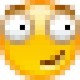12:22 AM
7I'm trying to understand how to properly structure a C++ project by using CMake, googletest, and gcov for test coverage. I would like to build a general CMakeLists.txt that would work for any platform/compiler. This is my first attempt. However, if I try to build the project and then run lcov (to...12:52 AM
5What is known about the classification of knots in a solid torus $S^1 \times D^2$? Is enumerating them a reasonable problem? Do we get a similar classification as for knots in $S^3$? Ideally there would be a simple description of the Seifert fibered knots in $S^1 \times D^2$ like for prime non-s...1:19 AM
2As I see it, $p$-adic integers work very similar to formal power series over $x$ (e g. with regards to Hensel lifting). When it comes to computing $\log P(x)$, one may use the formula $$(\log P)' = \frac{P'}{P}$$ to compute the expansion of the logarithm of $P(x)$ with $P(0)=1$ as  \log P \eq...

12 hours later…1:40 PM
0I want to knit the following code into an html document. The code runs fine in Rstudio but fails when I attempt to knit - what am I missing here? load(url("http://www.stanford.edu/~hastie/ElemStatLearn/datasets/ESL.mixture.rda")) names(ESL.mixture) attach(ESL.mixture) plot(x,col=y+2,pch=c(17,19)...

6 hours later…7:40 PM
0the data.frame is like: |col_1| |------| |A | |B | |B | |A | |A | The expected output should be: |col_A |col_B | |---------|-------| |A |NA | |NA |B | |A |NA | |NA |B | |A |NA | |A |NA | I can do this manually in excel but is there a concise way in R t...8:28 PM
0How would you create an HTML code for an (RSS Feed Like) text box that automatically creates next box in ascending order? It is Something Similar to TT-RSS Example: BOX3 (newest): HELLO TEXT 3 (DATE DD:MM:YYYY) BOX2: HELLO TEXT 2 (DATE DD:MM:YYYY) BOX1: HELLO TEXT 1 (DATE DD:MM:YYYY)

2 hours later…10:08 PM
12Given two positive integer fractions \$x\$ and \$y\$ such that \$x < y\$, give the fraction \$z\$ with the smallest positive integer denominator such that it is between \$x\$ and \$y\$. For example \$x=2/5\$, \$y=4/5\$, the answer is \$1/2\$. Other fractions such as \$3/5\$ are also in between t...

1 hour later…11:34 PM
4My knowledge of automorphic forms basically comes from Lang's $SL_2$ and Borel's automorphic forms on $SL_2$. I try to understand them from representation theory. For example, an integral weight $k$ automorphic form corresponding to the $k$-th character eigenspace for the right $SO_2$ action on \$...